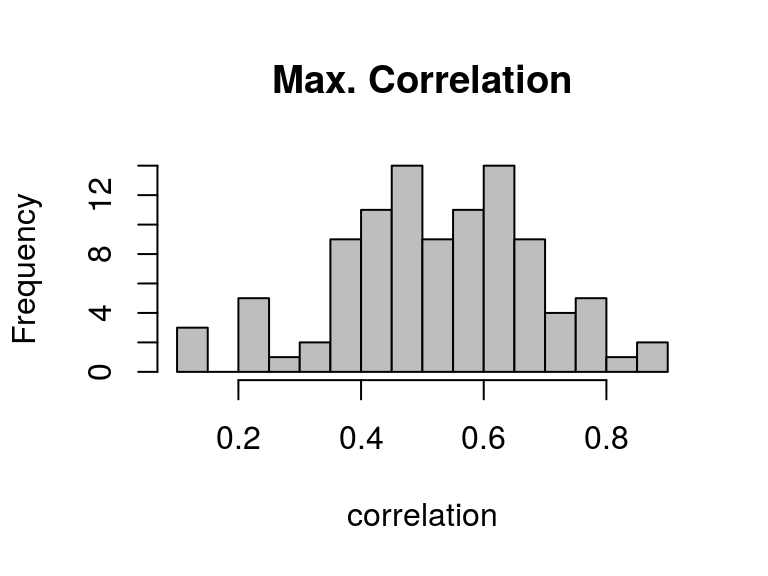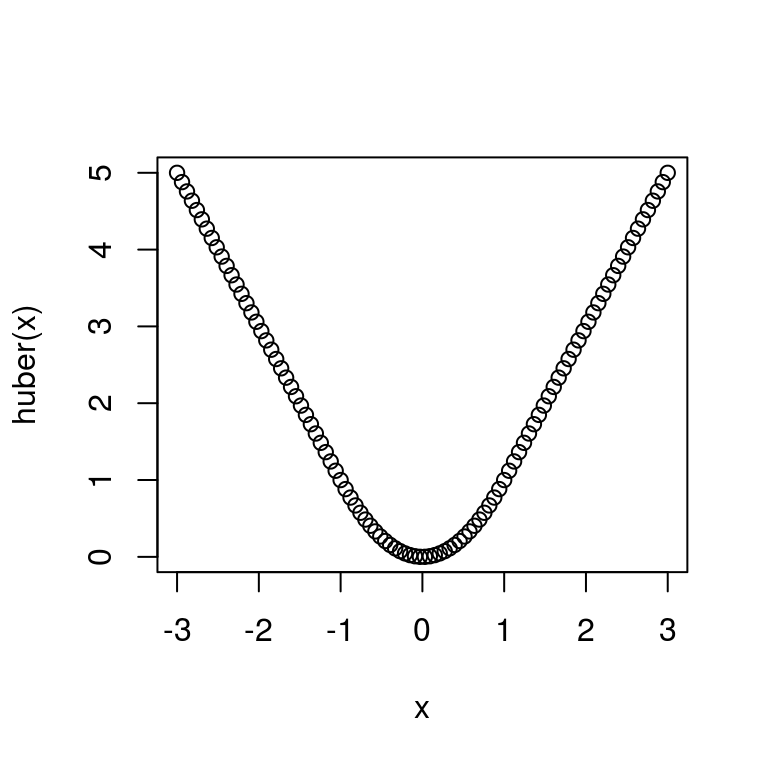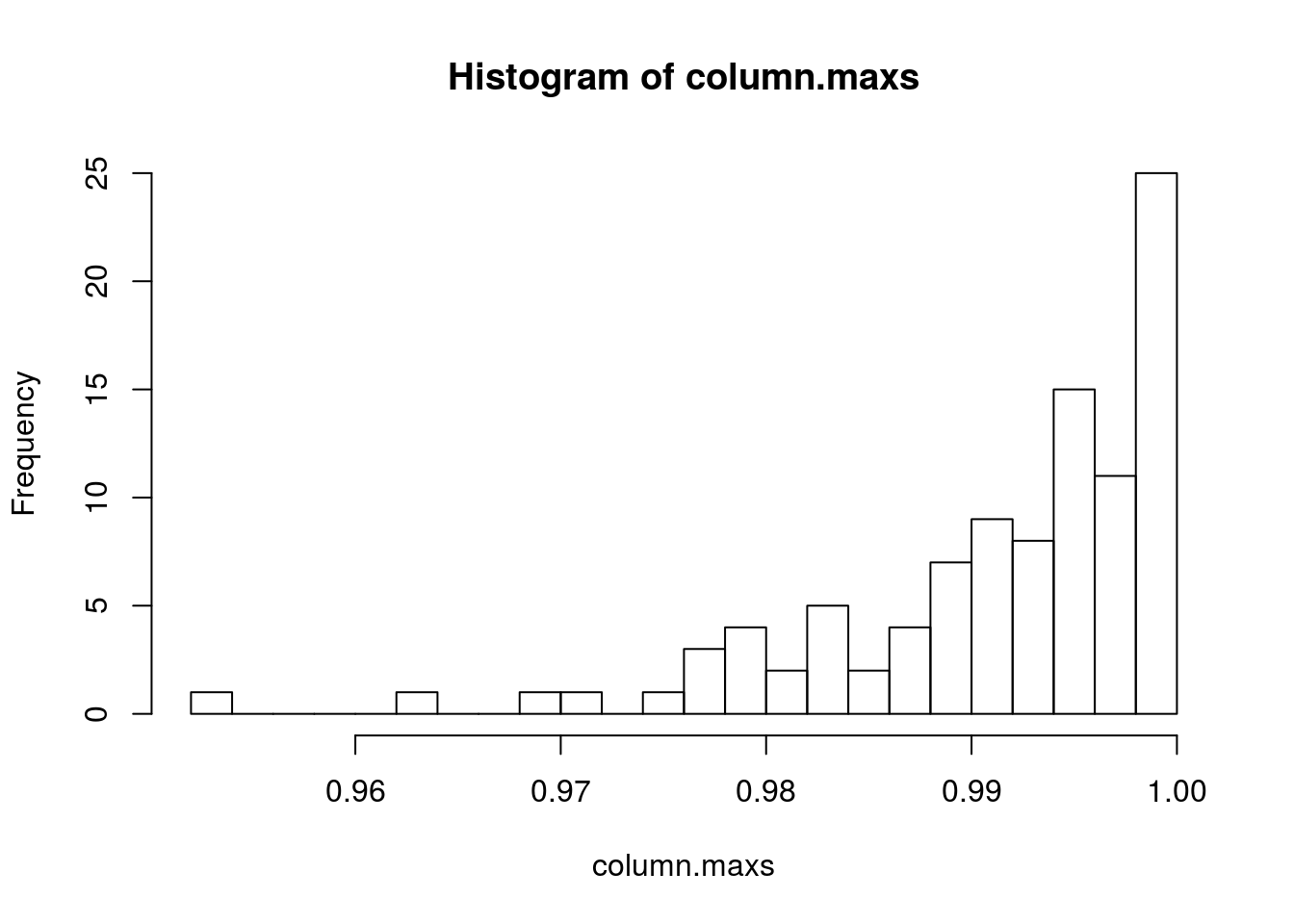# Style

• You will comment your code
• Comment every function with purpose, inputs, outputs
• You will use meaningful names
• You will indent code blocks (Control-I)

# Calculate quantiles of the Pareto distribution
# Inputs: desired quantile (p)
# exponent of the distribution (exponent)
# lower threshold of the distribution (threshold)
# Outputs: the pth quantile
qpareto.1 <- function(p, exponent, threshold) {
q <- threshold*((1-p)^(-1/(exponent-1)))
return(q)
}
• You ought to avoid calling your main data set data
• vague
• often the name of arguments in e.g. model-fitting functions
• both of those make it hard to trace what’s going on
#Excerpt from HW1 solutions
value.median.mean <- lm(Median_house_value ~ Median_household_income
+ Mean_household_income, data=data)
• Makes you feel crazy

A treat: The following is code from a prominent statistician:

### CENSORED TO PROTECT THE INNOCENT ###

# Functions

• Use functions to tie together related commands so they can be repeated

Example: Suppose that we want to easily compute the quantiles of the Pareto distribution. The density function is: $f(x;\alpha,x_0) = \begin{cases} \frac{\alpha-1}{x_0}\left(\frac{x}{x_0}\right)^{-\alpha} & x \ge x_0\\ 0 & x < x_0 \end{cases}$ and the quantile function is $Q(p; \alpha,x_0) = x_0(1-p)^{-\frac{1}{\alpha-1}}$

# Calculate quantiles of the Pareto distribution
# Inputs: desired quantile (p)
# exponent of the distribution (exponent)
# lower threshold of the distribution (threshold)
# Outputs: the pth quantile
qpareto.1 <- function(p, exponent, threshold) {
q <- threshold*((1-p)^(-1/(exponent-1)))
return(q)
}

# alpha = 2.33, x_0 = 6e8, p = 0.5
6e8 * (1-0.5)^(-1/(2.33-1))
##  1010391288
# alpha = 2.33, x_0 = 6e8, p = 0.4
6e8 * (1-0.4)^(-1/(2.33-1))
##  880957225
# alpha = 2.5, x_0 = 1e6, p = 0.92
1e6 * (1-0.92)^(-1/(2.5-1))
##  5386087

we can say

qpareto.1(p=0.5, exponent=2.33, threshold=6e8)
##  1010391288
qpareto.1(p=0.4, exponent=2.33, threshold=6e8)
##  880957225
qpareto.1(p=0.92, exponent=2.5, threshold=1e6)
##  5386087

Much harder to make mistakes!

• Arguments: what does the function need to know?
• Default values let you omit arguments that don’t change often
• Default values can always be over-ridden
# Calculate quantiles of the Pareto distribution
# Inputs: desired quantile (p)
# exponent of the distribution (exponent)
# lower threshold of the distribution (threshold)
# flag for whether to give lower or upper quantiles (lower.tail)
# Outputs: the pth quantile
qpareto.2 <- function(p, exponent, threshold, lower.tail=TRUE) {
if(lower.tail==FALSE) {
p <- 1-p
}
q <- threshold*((1-p)^(-1/(exponent-1)))
return(q)
}

qpareto.2(p=0.92, exponent=2.5, threshold=1e6)
##  5386087
qpareto.2(p=0.92, exponent=2.5, threshold=1e6, lower.tail=FALSE)
##  1057162
• No-argument functions can be useful for simulation
# (Unrelated to the quantile functions)
# Function to return the biggest correlation of 5 length-10 Gaussian vectors
# Takes no arguments, so it can be used in a simulation
random.correlation.max = function(){
#Draw a 10x5 matrix of Gaussians
X = matrix(rnorm(50),nrow=10,ncol=5)
#Compute the correlation matrix
cor.matrix = cor(X)
#Find the biggest entry, making sure to avoid the diagonal (which is all 1)
return(max(cor.matrix[upper.tri(cor.matrix)]))
}

#Draw 100 samples and plot them
hist(replicate(100,random.correlation.max()),20,col='grey', main="Max. Correlation",xlab='correlation')• ... indicates pass-through arguments. You won’t use this very often, but many R functions do.
• Return value
• Try to be explicit about what you return
• Use c() or list() to return more than one thing (We will see some examples of this)

# When should we write functions?

• When we’re repeating the same thing multiple times
• When we’re writing very similar code over and over
• When we’re breaking down a big job into smaller ones
• When we’re told to

Example: From Solutions to Homework 1. You were asked to make several maps of your residuals. The following function was modified from last term.

# Set up a function to make maps
# "Terrain" color-levels set based on quantiles of the variable being plotted
# Inputs: vector to be mapped over the data frame; number of levels to
# use for colors; name of the longitude coordinate; name of the lattitude
# coordinate; data frame to take coordinates from; other plotting arguments
# Outputs: invisibly, list giving cut-points and the level each observation
# was assigned
mapper <- function(z, levels, x="LONGITUDE", y="LATITUDE", data,
legend.loc="topright", ncol=1, ...) {
# Which quantiles do we need?
probs <- seq(from=0, to=1, length.out=(levels+1))
# What are those quantiles?
z.quantiles <- quantile(z, probs)
# Assign each observation to its quantile
z.categories <- cut(z, z.quantiles, include.lowest=TRUE)
# Make up a color scale
ncol=ncol, pch=19)
invisible(list(quantiles=z.quantiles, categories=z.categories))
}

# Chaining functions together

• Divide the big job (“come up with a confidence band”) into smaller parts
• Write a function for each part
• Make sure the outputs of the early steps can be used as inputs to the later steps
• Recurse if need be: the sub-functions spawn sub-sub-functions, etc.
• We can often re-use the sub-functions (e.g., resample())

Example I work on a statistics problem in inference for networks/graphs. Suppose that I want to run a hypothesis test about an edge in the graph. I might need:

• A function that carries out the test. It calls:
• A function that can draw a sample from a particular null distribution. It calls:
• A function that can check the validity of a particular sample.

This is helpful for many reasons:

• Many different tests use this null distribution
• The validity check shows up in many other problems
• I can debug each piece individually, starting from the bottom
• I can have a set of tests for each piece, so I notice when I break it

# Calling functions from other functions

It is often convenient to call functions from other functions, as we build functionality. As a simple example, we could have avoided replicating the quantile code from qpareto.1 in qpareto.2 as follows.

# Calculate quantiles of the Pareto distribution
# Inputs: desired quantile (p)
# exponent of the distribution (exponent)
# lower threshold of the distribution (threshold)
# flag for whether to give lower or upper quantiles (lower.tail)
# Outputs: the pth quantile
qpareto.3 <- function(p, exponent, threshold, lower.tail=TRUE) {
if(lower.tail==FALSE) {
p <- 1-p
}
q <- qpareto.1(p, exponent, threshold)
return(q)
}

This calls qpareto.1 to do the actual calculation, after making corrections for the lower.tail argument.

This is very useful! You will often be working with several intertwined functions at the same time. We should be very careful to understand what happens.

A very simple example:

# A very simple function that adds one to its argument
x+1
}

}

add.stuff(3)
##  4
# A terrible job rewriting add.one
x+2
}

#Now what?
add.stuff(3)
##  5

The add.stuff function looks for add.one each time it is called. If add.one has changed, it uses the new version of the function. It does not save a snapshot at the time add.stuff is defined.

This is great, since it lets you easily edit all your functions and have the changes propagate. However, you do need to be aware and careful.

# Debugging

Eventually, you will break something.

• Problem: the final piece of code you write gives an incomprehensible error message
• Idea: Carefully characterize the bug, then narrow down where it could be until the the problem is obvious.
• Strategy: make sure all the lower-level pieces are working properly
• Start debugging from the bottom
• If all the small bits work on their own, the bug comes when you put them together
• Use test cases
• “What should this do if it works?”
• “What should this not do if it’s broken?”
• When you meet a new bug, write a new test
• “How do I know if this is fixed?”
• Test could be very minimal: “It runs without crashing”
• traceback(): Show all the calls leading to the last error
• Look for the last line of code you wrote
• This helps localize where things went wrong
• browser(): A convenient way to mess around inside a function -When called within a function, gives you an R shell there -Avoids problems with local variables disappearing
• A favorite line: Executes [Dangerous Expression] and calls browser() if there is an error. Great for seeing problems that only happen sometimes inside of for loops inside of functions.

tryCatch({[Dangerous Expression]},warning=function(w){browser()},
error=function(e){browser()})

Example: Suppose that I want to sample a Pareto random variable. I know that if $$F(x)$$ is the CDF for a distribution and $$U$$ is a uniform random variable, then $$F^{-1}(U)$$ has the F distribution.

First, for good practice, define a version of the quantile function that validates inputs

# Calculate quantiles of the Pareto distribution
# Inputs: desired quantile (p)
# exponent of the distribution (exponent)
# lower threshold of the distribution (threshold)
# flag for whether to give lower or upper quantiles (lower.tail)
# Outputs: the pth quantile
qpareto.4 <- function(p, exponent, threshold, lower.tail=TRUE) {
#browser()
stopifnot(p >= 0, p <= 1, exponent > 1, threshold > 0)
q <- qpareto.3(p,exponent,threshold,lower.tail)
return(q)
}
#Try it with a bad input
qpareto.4(p=0.92,exponent=2.5,threshold=-1,lower.tail=FALSE)

Now we can define a random pareto function. This version is (intentionally) buggy!

# Generate random numbers from the Pareto distribution
# Inputs: number of random draws (n)
# exponent of the distribution (exponent)
# lower threshold of the distribution (threshold)
# Outputs: vector of random numbers
rpareto <- function(n,exponent,threshold) {
x <- vector(length=n)
for (i in 1:n) {
x[i] <- qpareto.4(p=runif(1),exponent=exponent,threshold=threshold)
}
return(x)
}

We can run it and get an error

# If we run this block in Rmarkdown, it will break our document
# Run this in an R session.
rpareto(10)
rpareto(n=10,exponent=2.5,threshold=1)

# Avoid iteration

• Iterating in R is often quite slow
• R has lots of ways to avoid iteration
• This is often faster! And easier to follow!

Compare:

# Generate random numbers from the Pareto distribution
# Inputs: number of random draws (n)
# exponent of the distribution (exponent)
# lower threshold of the distribution (threshold)
# Outputs: vector of random numbers
rpareto.1 <- function(n,exponent,threshold) {
x <- vector(length=n)
for (i in 1:n) {
x[i] <- qpareto.4(p=runif(1),exponent=exponent,threshold=threshold)
}
return(x)
}

# Generate random numbers from the Pareto distribution
# Inputs: number of random draws (n)
# exponent of the distribution (exponent)
# lower threshold of the distribution (threshold)
# Outputs: vector of random numbers
rpareto.2 <- function(n,exponent,threshold) {
x = replicate(n, qpareto.4(p=runif(1),exponent=exponent,threshold=threshold))
return(x)
}

system.time(rpareto.1(10000,exponent=2.5,threshold=1))
##    user  system elapsed
##   0.243   0.000   0.243
system.time(rpareto.2(10000,exponent=2.5,threshold=1))
##    user  system elapsed
##   0.377   0.000   0.409

We could try vectorizing our function better:

#Our qpareto1 functions can handle vector p arguments just fine.
qpareto.4(c(.1,.2,.3), 2.5, 1)
##  1.072766 1.160397 1.268434
# Generate random numbers from the Pareto distribution
# Inputs: number of random draws (n)
# exponent of the distribution (exponent)
# lower threshold of the distribution (threshold)
# Outputs: vector of random numbers
rpareto.3 <- function(n,exponent,threshold) {
x = qpareto.4(p=runif(n), exponent=exponent, threshold=threshold)
return(x)
}
system.time(rpareto.3(10000,exponent=2.5,threshold=1))
##    user  system elapsed
##   0.003   0.000   0.002

Other approaches to removing iteration

• use ifelse to do conditional things to entire vectors

# Calculate Huber's loss function
# Input: vector of numbers x
# Return: x^2 for |x|<1, 2|x|-1 otherwise
huber <- function(x) {
return(ifelse(abs(x) <= 1, x^2, 2*abs(x)-1))
}
x = seq(from=-3,to=3,length.out=100)
plot(x,huber(x))• use matrix operations

# Get some long vectors
n = 100000
a = rnorm(n)
b = rnorm(n)
# Find the inner product with a for loop
system.time({
total = 0
for(i in 1:length(a)){total = total + a[i]*b[i]}
})
##    user  system elapsed
##   0.140   0.000   0.153
# Find the inner product with matrix operations
system.time({
a %*% b #R handles vector dimensions in a funny way here.  Read ?matmult
})
##    user  system elapsed
##   0.001   0.000   0.001
• use vector arithmetic

# Get some long vectors
n = 100000
a = rnorm(n)
b = rnorm(n)

# Find the sum with a for loop, don't preallocate memory
system.time({
total = numeric(0) #Don't actually allocate any space
for(i in 1:length(a)){total[i] = a[i]+b[i]}
})
##    user  system elapsed
##  21.871   0.016  21.983
# Find the sum with a for loop, allocate memory all at once
system.time({
total = numeric(n) #Allocate space ahead of time
for(i in 1:length(a)){total[i] = a[i]+b[i]}
})
##    user  system elapsed
##   0.183   0.000   0.183
# Find the inner product with matrix operations
system.time({
a + b #R handles vector dimensions in a funny way here.  Read ?matmult
})
##    user  system elapsed
##   0.000   0.000   0.001
• use apply to apply a function to rows or columns of a matrix. Use sapply to apply a function to every element of a vector.

#Make a 100x100 matrix of uniform [0,1] variables
X = matrix(runif(10000),nrow=100,ncol=100)
#Find the maximum of each column
column.maxs = apply(X, MARGIN = 2, FUN = max)
#Plot a histogram.  Do you know the distribution?
hist(column.maxs,20)A sidenote on matrix arithmetic and subsetting

When subsetting a matrix, we expect R to return another matrix.

#Make a matrix
X = matrix(rnorm(12),nrow=4,ncol=3)
X
##            [,1]       [,2]        [,3]
## [1,] -1.5156656 -0.7527156  0.27717781
## [2,]  0.3802144  0.8397816 -0.90298396
## [3,]  0.5414839 -0.7360646  0.06724232
## [4,]  0.2960943 -0.7469858  0.45159979
#We would expect the following to all return 3x3 matrices
t(X) %*% X
##            [,1]       [,2]       [,3]
## [1,]  2.8226820  0.8404169 -0.5933096
## [2,]  0.8404169  2.3715927 -1.3537787
## [3,] -0.5933096 -1.3537787  1.1006715
t(X[1:3,]) %*% X[1:3,]
##            [,1]      [,2]       [,3]
## [1,]  2.7350101  1.061595 -0.7270257
## [2,]  1.0615951  1.813605 -1.0164401
## [3,] -0.7270257 -1.016440  0.8967291
t(X[1:2,]) %*% X[1:2,]
##            [,1]       [,2]       [,3]
## [1,]  2.4418053  1.4601622 -0.7634363
## [2,]  1.4601622  1.2718139 -0.9669454
## [3,] -0.7634363 -0.9669454  0.8922076
t(X[1,]) %*% X[1,]
##          [,1]
## [1,] 2.940651

What went wrong?

    dim(X)
##  4 3
    dim(X[1:2,])
##  2 3
    dim(X[1,])
## NULL

When we choose a subset with at least one dimension 1, R drops those dimensions! For a 2-D matrix, this becomes a 1-D vector! Many functions treat a vector very differently.

dim(X)
##  4 3
length(X)
##  12
dim(X[,1])
## NULL
length(X[,1])
##  4

To fix this, we can specify that the additional dimension should not be dropped.

dim(X[,1,drop=FALSE])
##  4 1
length(X[,1,drop=FALSE])
##  4
t(X[1,,drop=FALSE]) %*% X[1,,drop=FALSE]
##            [,1]       [,2]        [,3]
## [1,]  2.2972423  1.1408652 -0.42010889
## [2,]  1.1408652  0.5665808 -0.20863607
## [3,] -0.4201089 -0.2086361  0.07682754

# Do not be afraid of iteration

• Some jobs are just inherently iterative
• Use it when that’s what you need to do
• If you’re storing stuff as you go, create the variables which you’re storing into outside the loop
sim.ar <- function(n,x1,phi=0,sd=1) {
x <- vector(length=n)
x <- x1
for (t in 2:n) {
x[t] <- phi*x[t-1]+rnorm(1,mean=0,sd=sd)
}
return(x)
}

# Returning vectors and lists

A function can return one thing. Often you want to return more than one value. - For several values of the same type, you can return a vector - For several different sorts of values, you can return a list. You can even assign names!

For an example of returning a list, we return to the random correlation matrix example

# Function to return the correlation matrix of 5 length-10 Gaussian vectors
random.correlation.matrix = function(){
#Draw a 10x5 matrix of Gaussians
X = matrix(rnorm(50),nrow=10,ncol=5)
#Compute the correlation matrix
cor.matrix = cor(X)
cor.matrix
}
#Function to compute summaries of a correlation matrix
matrix.extremes = function(M){
#Extract the off-diagonal entries
off.diagonal = M[upper.tri(M)]
#Return the largest and smallest off-diagonal entries
return(list(max = max(off.diagonal), min = min(off.diagonal)))
}
matrix.extremes(random.correlation.matrix())
## $max ##  0.6462206 ## ##$min
##  -0.6484274
replicate(5,matrix.extremes(random.correlation.matrix()))
##     [,1]       [,2]       [,3]       [,4]       [,5]
## max 0.5685958  0.5498803  0.4050465  0.4095796  0.729471
## min -0.3054157 -0.2361346 -0.5031285 -0.6958686 -0.3911191

For an example of returning a vector, we look at our rpareto function from earlier:

# Generate random numbers from the Pareto distribution
# Inputs: number of random draws (n)
# exponent of the distribution (exponent)
# lower threshold of the distribution (threshold)
# Outputs: vector of random numbers
rpareto.3 <- function(n,exponent,threshold) {
x = qpareto.4(p=runif(n), exponent=exponent, threshold=threshold)
return(x) #If n>1, this is a vector!
}

#This will return a vector of 10 things:
rpareto.3(10,2.5,1)
##   1.022777 1.287552 1.280614 2.908297 2.329300 2.340561 2.386480
##   2.973543 1.347765 2.358582